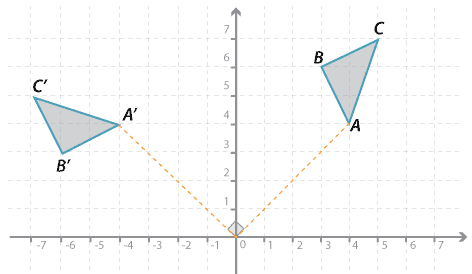### Rotating by 90 ° in an anticlockwise direction about the origin

In the diagram below, triangle $$ABC$$ is rotated 90 ° in an anticlockwise direction about the origin:

$$A(4,4) \rightarrow A^\prime (−4,4); B(3,6) \rightarrow B^\prime (−6,3); C(5,7) \rightarrow C^\prime (−7,5)$$.

In general, $$P(x,y) \rightarrow P^\prime (−y,x)$$.Detailed description

#### A rule for the rotation of 90 ° in an anticlockwise direction.

If we wish to work out a rule for the rotation of 90 ° in an anticlockwise direction, say, about $$D$$(7, 6), we can follow the procedure shown below.

Translate $$A$$(4, 4) 7 units to the left and 6 units down:

$$(4, 4) \rightarrow (4 − 7, 4 − 6) = (−3, −2)$$

Rotate around the origin by 90 ° in an anticlockwise direction:

$$(−3, −2) \rightarrow (2, −3)$$

Now translate back:

$$(2, −3) \rightarrow (2 + 7, −3 + 6) = (9, 3)$$

In general, $$(x, y) \rightarrow(x − 7, y − 6) \rightarrow (6 − y, x − 7) \rightarrow (13 − y, x − 1)$$.